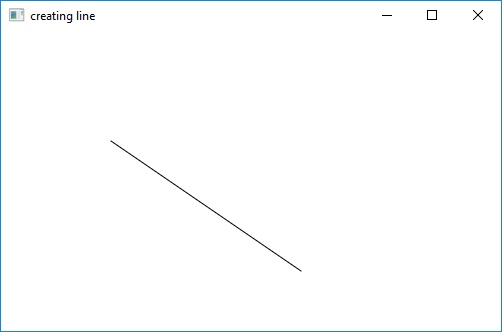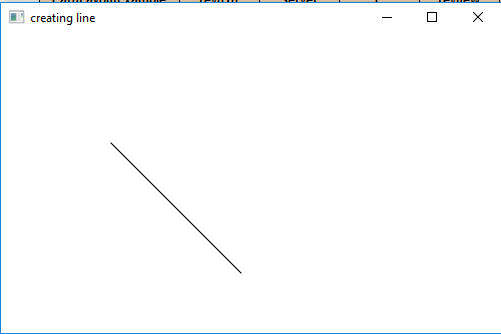# JavaFX | Line with examples

Line is a part of JavaFX. The Line class represents a line in a 2D space.

Constructor for the class:

1. Line(): Creates a new instance for line
2. Line(double startX, double startY, double endX, double endY): Creates a new Line with specified staring and ending point

Commonly Used Methods:

Method Explanation
getEndX() returns the x coordinate for the end point
getEndY() returns the y coordinate for the end point
getStartX() returns the x coordinate for the start point
getStartY() returns the y coordinate for the start point
setEndX(double value) sets the x coordinate for the end point
setEndY(double value) sets the y coordinate for the end point
setStartX(double value) sets the x coordinate for the start point
setStartY(double value) sets the y coordinate for the start point

Below programs illustrate the Line class:

1. Java program to create a line with starting and ending coordinates passed as arguments: This program creates a Line indicated by the name line( start point and the end point is passed as arguments). The Line will be created inside a scene, which in turn will be hosted inside a stage. The function setTitle() is used to provide title to the stage. Then a Group is created, and the line is attached. The group is attached to the scene. Finally, the show() method is called to display the final results.

 `// Java program to create a line with starting ` `// and ending coordinates passed as arguments ` `import` `javafx.application.Application; ` `import` `javafx.scene.Scene; ` `import` `javafx.scene.shape.DrawMode; ` `import` `javafx.scene.layout.*; ` `import` `javafx.event.ActionEvent; ` `import` `javafx.scene.shape.Line; ` `import` `javafx.scene.control.*; ` `import` `javafx.stage.Stage; ` `import` `javafx.scene.Group; ` ` `  `public` `class` `line_0 ``extends` `Application { ` ` `  `    ``// launch the application ` `    ``public` `void` `start(Stage stage) ` `    ``{ ` `         `  `        ``// set title for the stage ` `        ``stage.setTitle(``"creating line"``); ` ` `  `        ``// create a line ` `        ``Line line = ``new` `Line(``10``.0f, ``10``.0f, ``200``.0f, ``140``.0f); ` ` `  `        ``// create a Group ` `        ``Group group = ``new` `Group(line); ` ` `  `        ``// translate the line to a position ` `        ``line.setTranslateX(``100``); ` `        ``line.setTranslateY(``100``); ` ` `  `        ``// create a scene ` `        ``Scene scene = ``new` `Scene(group, ``500``, ``300``); ` ` `  `        ``// set the scene ` `        ``stage.setScene(scene); ` ` `  `        ``stage.show(); ` `    ``} ` ` `  `    ``// Main Method ` `    ``public` `static` `void` `main(String args[]) ` `    ``{ ` `        ``// launch the application ` `        ``launch(args); ` `    ``} ` `} `

Output:2. Java program to create a line with starting and ending coordinates set using function setStartX(), setStartY()setEndX(), setEndY() function: This program creates a Line indicated by the name line(start point and end point is set using setEndX(), setEndY(), setStartX(), setStartY() function). The Line will be created inside a scene, which in turn will be hosted inside a stage. The function setTitle() is used to provide title to the stage. Then a Group is created, and the line is attached.The group is attached to the scene. Finally, the show() method is called to display the final results.

 `// Java program to create a line with starting  ` `// and ending coordinates set using function  ` `// setStartX(), setStartY() setEndX(), ` `// setEndY() function ` `import` `javafx.application.Application; ` `import` `javafx.scene.Scene; ` `import` `javafx.scene.shape.DrawMode; ` `import` `javafx.scene.layout.*; ` `import` `javafx.event.ActionEvent; ` `import` `javafx.scene.shape.Line; ` `import` `javafx.scene.control.*; ` `import` `javafx.stage.Stage; ` `import` `javafx.scene.Group; ` ` `  `public` `class` `line_1 ``extends` `Application { ` ` `  `    ``// launch the application ` `    ``public` `void` `start(Stage stage) ` `    ``{ ` `        ``// set title for the stage ` `        ``stage.setTitle(``"creating line"``); ` ` `  `        ``// create a line ` `        ``Line line = ``new` `Line(); ` ` `  `        ``// set staring position ` `        ``line.setStartX(``10``.0f); ` `        ``line.setStartY(``10``.0f); ` ` `  `        ``// set ending position ` `        ``line.setEndX(``140``.0f); ` `        ``line.setEndY(``140``.0f); ` ` `  `        ``// create a Group ` `        ``Group group = ``new` `Group(line); ` ` `  `        ``// translate the line to a position ` `        ``line.setTranslateX(``100``); ` `        ``line.setTranslateY(``100``); ` ` `  `        ``// create a scene ` `        ``Scene scene = ``new` `Scene(group, ``500``, ``300``); ` ` `  `        ``// set the scene ` `        ``stage.setScene(scene); ` ` `  `        ``stage.show(); ` `    ``} ` ` `  `    ``// Main Method ` `    ``public` `static` `void` `main(String args[]) ` `    ``{ ` `         `  `        ``// launch the application ` `        ``launch(args); ` `    ``} ` `} `

Output:Note: The above programs might not run in an online IDE. Please use an offline compiler.

My Personal Notes arrow_drop_upCheck out this Author's contributed articles.

If you like GeeksforGeeks and would like to contribute, you can also write an article using contribute.geeksforgeeks.org or mail your article to contribute@geeksforgeeks.org. See your article appearing on the GeeksforGeeks main page and help other Geeks.

Please Improve this article if you find anything incorrect by clicking on the "Improve Article" button below.

Article Tags :

Be the First to upvote.

Please write to us at contribute@geeksforgeeks.org to report any issue with the above content.